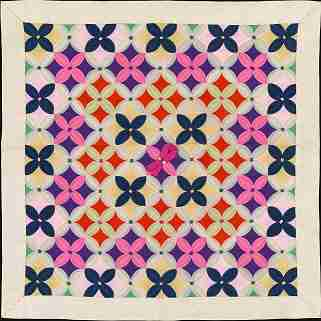# Definition of PatternA pattern is an arrangement of numbers or geometric objects according to a given rule or set of rules.

For example, the navy, pink and yellow flowers in the quilt in the picture are arranged in a pattern.

A mathematical example is the number sequence, $2,4,8,16,32,\dots$. To form this number pattern, you need to follow the rule to "start at $2$ and double the number each time".

### Description

The aim of this dictionary is to provide definitions to common mathematical terms. Students learn a new math skill every week at school, sometimes just before they start a new skill, if they want to look at what a specific term means, this is where this dictionary will become handy and a go-to guide for a student.

### Audience

Year 1 to Year 12 students

### Learning Objectives

Learn common math terms starting with letter P

Author: Subject Coach
You must be logged in as Student to ask a Question.